Announcements
Applications are open for the 2024 Qlik Luminary Program. Apply by December 15 here.
cancel
Showing results for
Did you mean:Anonymous
Not applicable

## A difficult nut to crack!

Hi Experts,

I'm trying to present data in a straight table in a certain way. Below is the table I have.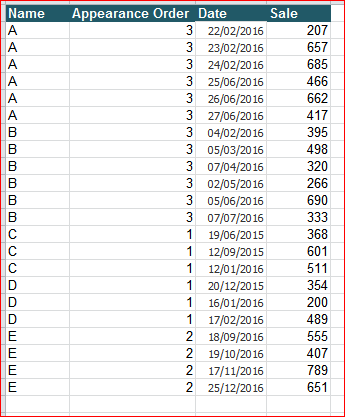The Table has Name, Appearance Order, Date and Sale value. I want to present this in a straight table using the Appearance order. For example, the Appearance order is 3 for A, so I should be able to see only last 3 Sale and date values for Name A. I want to present this data in like this in a straight table: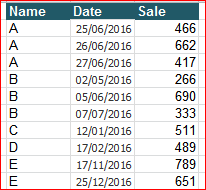Is there anyway we can do this? Either at the script level or within Qlik at expression level?

Thanks

1 Solution

Accepted SolutionsMVP

You tried this

=Aggr(If(Date >= Only(Total <Name> Aggr(If(Max(TOTAL <Name> Date) = Date, Above(Date, [Appearance Order]-1)), Name, Date)), Date), Name, Date)

I want you to try this

=Aggr(If(Date >= Only(Total <Name> Aggr(If(Max(TOTAL <Name> Date) = Date, Above(Date, [Appearance Order]-1)), Name, (Date,(numeric)))), Date), Name, Date)

See the difference?

21 RepliesMVP

Check this outDimensions

Name

=Aggr(If(Date >= Only(Total <Name> Aggr(If(Max(TOTAL <Name> Date) = Date, Above(Date, [Appearance Order]-1)), Name, Date)), Date), Name, Date)

Expression

Sum(Sale)MVP

But 01/03/2016 is smaller than 26/06/2016? Why do you want this to show up or did you mean 01/03/2017?MVP

For 2017 it seems to be working, but not sure I understand the logic for 2016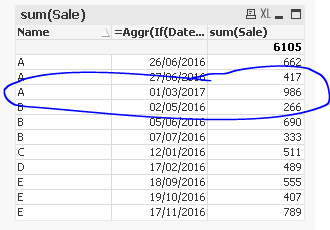Anonymous
Not applicable
Author

Apologies Sunny. It was my mistake. Please ignore my last post but I've run into another trouble. I'm attaching a file where I've just replaced the dates but for some reason Appearance Order isn't working.

This is my new table,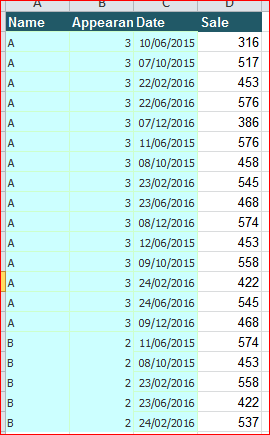The result should be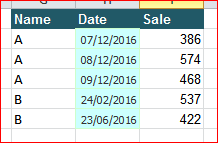But the application is showing...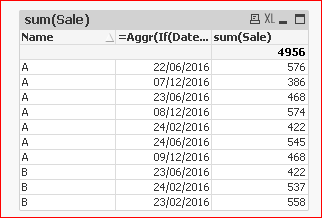All I did was change the data. Do you think this is because of date format?MVP

I hope you are using QlikView 12 or Qlik Sense, because if you are not, then you might have to make changes within the script to make this work. For QV12 or Qlik Sense, you can try this

=Aggr(If(Date >= Only(Total <Name> Aggr(If(Max(TOTAL <Name> Date) = Date, Above(Date, [Appearance Order]-1)), Name, (Date,(numeric)))), Date), Name, Date)Anonymous
Not applicable
Author

Hi Sunny thanks for quick reply. Sorry I didn't understand what you mean by numeric? Can you please elaborate?MVP

This was a new functionality added in QV12 which allowed the Aggr() function to be sorted in other than just load order. Previous to QV12, Aggr() function always sorted in load order (which is what it is doing today and messing your expression).Anonymous
Not applicable
Author

Hi Sunny,

I just tried the same expression in Qlik Sense v 3.2 and getting the same result for some reason.

I'm using this expression

=Aggr(If(Date >= Only(Total <Name> Aggr(If(Max(TOTAL <Name> Date) = Date, Above(Date, [Appearance Order]-1)), Name, Date)), Date), Name, Date)

Do you think I'll need to change the expression?I'll go through the link you sent me and study the new functionality. I do have slightly older version of QV but I've latest version of Qlik Sense. If it's showing me the same result in Qlik Sense as in QV then I might have to change the expression I guess.MVP

You tried this

=Aggr(If(Date >= Only(Total <Name> Aggr(If(Max(TOTAL <Name> Date) = Date, Above(Date, [Appearance Order]-1)), Name, Date)), Date), Name, Date)

I want you to try this

=Aggr(If(Date >= Only(Total <Name> Aggr(If(Max(TOTAL <Name> Date) = Date, Above(Date, [Appearance Order]-1)), Name, (Date,(numeric)))), Date), Name, Date)

See the difference?Tags
Community Browser